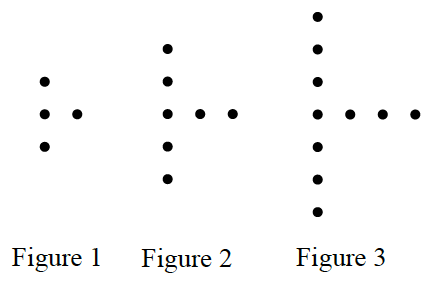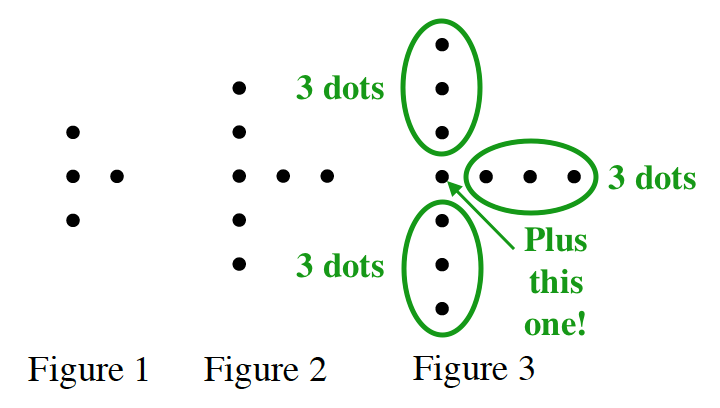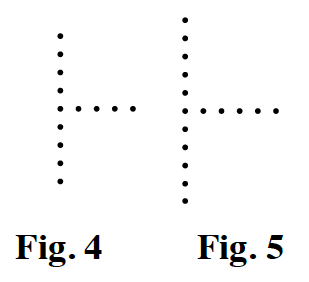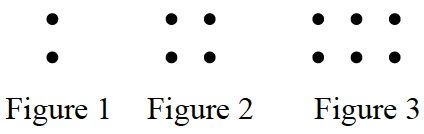Home > CC1 > Chapter 1 > Lesson 1.1.3 > Problem1-19

1-19.

Study the dot patterns in parts (a) and (b) below. Assume that each pattern continues to increase by the same number of dots and in the same locations for each figure. For each pattern, sketch the $4^{\text{th}}$ and $5^{\text{th}}$ figures. Then predict how many dots will be in the $100^{\text{th}}$ figure. 1-19a & 1-19b HW eTool (CPM). Homework Help ✎

1.Look at the vertical dots. How are they growing? What about the horizontal ones?

With each new figure, the vertical line gets one dot on the top and one dot on the bottom. The horizontal line gets one dot on the right side each time.

How can you find each figure number in the number of dots? Can you find groups of two dots in the second figure? Can you find groups of three dots in the third figure? Use this to help you with the $100^{\text{th}}$ figure.The $100^{\text{th}}$ figure will have $301$ dots

2.How many columns of two are in each figure? How many would you expect in figures 4 and 5? What about Figure 100?

3. For each pattern, explain how you made your prediction for the 100th figure.

Did you use numbers or diagrams? How do you know that your answer makes sense?

Use the eTool below to model more figures. Use what you learn to help answer the parts of the problem.
Click on the link at right for the full eTool version: CC1 1-19 HW eTool